# Creating MNE-Python data structures from scratch#

This tutorial shows how to create MNE-Python’s core data structures using an existing `NumPy array` of (real or synthetic) data.

We begin by importing the necessary Python modules:

```import numpy as np

import mne
```

## Creating `Info` objects#

The core data structures for continuous (`Raw`), discontinuous (`Epochs`), and averaged (`Evoked`) data all have an `info` attribute comprising an `mne.Info` object. When reading recorded data using one of the functions in the `mne.io` submodule, `Info` objects are created and populated automatically. But if we want to create a `Raw`, `Epochs`, or `Evoked` object from scratch, we need to create an appropriate `Info` object as well. The easiest way to do this is with the `mne.create_info` function to initialize the required info fields. Additional fields can be assigned later as one would with a regular `dictionary`.

To initialize a minimal `Info` object requires a list of channel names, and the sampling frequency. As a convenience for simulated data, channel names can be provided as a single integer, and the names will be automatically created as sequential integers (starting with `0`):

```# Create some dummy metadata
n_channels = 32
sampling_freq = 200  # in Hertz
info = mne.create_info(n_channels, sfreq=sampling_freq)
print(info)
```
```<Info | 7 non-empty values
ch_names: 0, 1, 2, 3, 4, 5, 6, 7, 8, 9, 10, 11, 12, 13, 14, 15, 16, 17, ...
chs: 32 misc
custom_ref_applied: False
highpass: 0.0 Hz
lowpass: 100.0 Hz
meas_date: unspecified
nchan: 32
projs: []
sfreq: 200.0 Hz
>
```

You can see in the output above that, by default, the channels are assigned as type “misc” (where it says `chs: 32 MISC`). You can assign the channel type when initializing the `Info` object if you want:

```ch_names = [f"MEG{n:03}" for n in range(1, 10)] + ["EOG001"]
info = mne.create_info(ch_names, ch_types=ch_types, sfreq=sampling_freq)
print(info)
```
```<Info | 7 non-empty values
ch_names: MEG001, MEG002, MEG003, MEG004, MEG005, MEG006, MEG007, MEG008, ...
chs: 3 Magnetometers, 6 Gradiometers, 1 EOG
custom_ref_applied: False
highpass: 0.0 Hz
lowpass: 100.0 Hz
meas_date: unspecified
nchan: 10
projs: []
sfreq: 200.0 Hz
>
```

If the channel names follow one of the standard montage naming schemes, their spatial locations can be automatically added using the `set_montage` method:

```ch_names = ["Fp1", "Fp2", "Fz", "Cz", "Pz", "O1", "O2"]
ch_types = ["eeg"] * 7
info = mne.create_info(ch_names, ch_types=ch_types, sfreq=sampling_freq)
info.set_montage("standard_1020")
```
Measurement date Unknown Unknown Unknown 10 points 7 EEG None Not available Not available 200.00 Hz 0.00 Hz 100.00 Hz

Note the new field `dig` that includes our seven channel locations as well as theoretical values for the three cardinal scalp landmarks.

Additional fields can be added in the same way that Python dictionaries are modified, using square-bracket key assignment:

```info["description"] = "My custom dataset"
print(info)
```
```<Info | 10 non-empty values
ch_names: Fp1, Fp2, Fz, Cz, Pz, O1, O2
chs: 7 EEG
custom_ref_applied: False
description: My custom dataset
dig: 10 items (3 Cardinal, 7 EEG)
highpass: 0.0 Hz
lowpass: 100.0 Hz
meas_date: unspecified
nchan: 7
projs: []
sfreq: 200.0 Hz
>
```

## Creating `Raw` objects#

To create a `Raw` object from scratch, you can use the `mne.io.RawArray` class constructor, which takes an `Info` object and a `NumPy array` of shape `(n_channels, n_samples)`. Here, we’ll create some sinusoidal data and plot it:

```times = np.linspace(0, 1, sampling_freq, endpoint=False)
sine = np.sin(20 * np.pi * times)
cosine = np.cos(10 * np.pi * times)
data = np.array([sine, cosine])

info = mne.create_info(
ch_names=["10 Hz sine", "5 Hz cosine"], ch_types=["misc"] * 2, sfreq=sampling_freq
)

simulated_raw = mne.io.RawArray(data, info)
simulated_raw.plot(show_scrollbars=False, show_scalebars=False)
```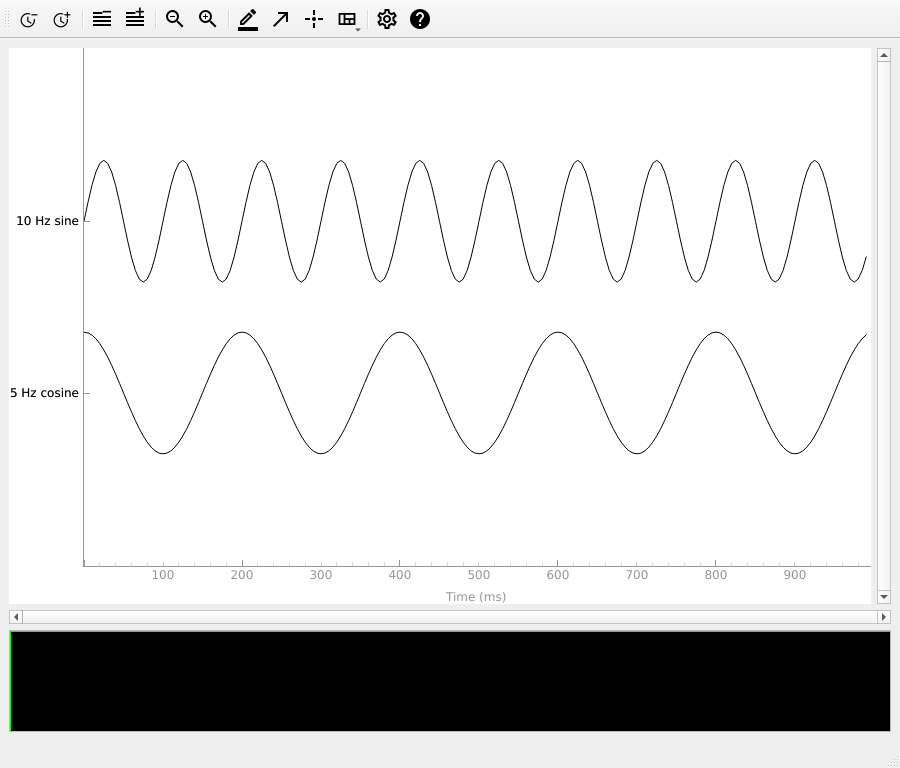```Creating RawArray with float64 data, n_channels=2, n_times=200
Range : 0 ... 199 =      0.000 ...     0.995 secs
```

## Creating `Epochs` objects#

To create an `Epochs` object from scratch, you can use the `mne.EpochsArray` class constructor, which takes an `Info` object and a `NumPy array` of shape ```(n_epochs, n_channels, n_samples)```. Here we’ll create 5 epochs of our 2-channel data, and plot it. Notice that we have to pass `picks='misc'` to the `plot` method, because by default it only plots data channels.

```data = np.array(
[
[0.2 * sine, 1.0 * cosine],
[0.4 * sine, 0.8 * cosine],
[0.6 * sine, 0.6 * cosine],
[0.8 * sine, 0.4 * cosine],
[1.0 * sine, 0.2 * cosine],
]
)

simulated_epochs = mne.EpochsArray(data, info)
simulated_epochs.plot(picks="misc", show_scrollbars=False, events=True)
```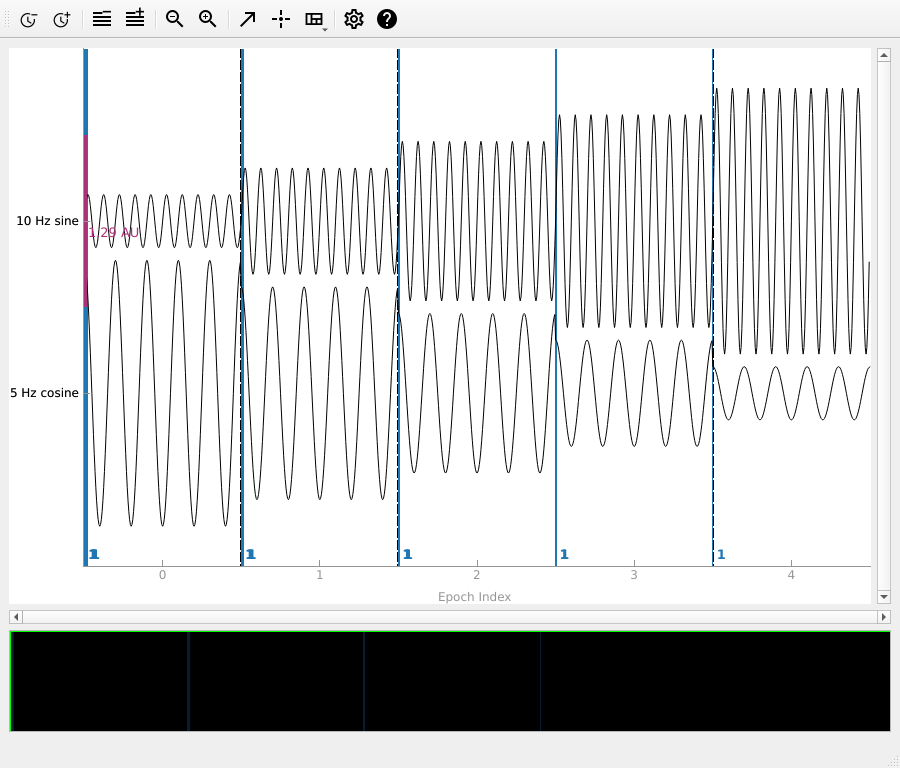```Not setting metadata
5 matching events found
No baseline correction applied
0 projection items activated
You seem to have overlapping epochs. Some event lines may be duplicated in the plot.
```

Since we did not supply an events array, the `EpochsArray` constructor automatically created one for us, with all epochs having the same event number:

```print(simulated_epochs.events[:, -1])
```
```[1 1 1 1 1]
```

If we want to simulate having different experimental conditions, we can pass an event array (and an event ID dictionary) to the constructor. Since our epochs are 1 second long and have 200 samples/second, we’ll put our events spaced 200 samples apart, and pass `tmin=-0.5`, so that the events land in the middle of each epoch (the events are always placed at time=0 in each epoch).

```events = np.column_stack(
(
np.arange(0, 1000, sampling_freq),
np.zeros(5, dtype=int),
np.array([1, 2, 1, 2, 1]),
)
)
event_dict = dict(condition_A=1, condition_B=2)
simulated_epochs = mne.EpochsArray(
data, info, tmin=-0.5, events=events, event_id=event_dict
)
simulated_epochs.plot(
picks="misc", show_scrollbars=False, events=events, event_id=event_dict
)
```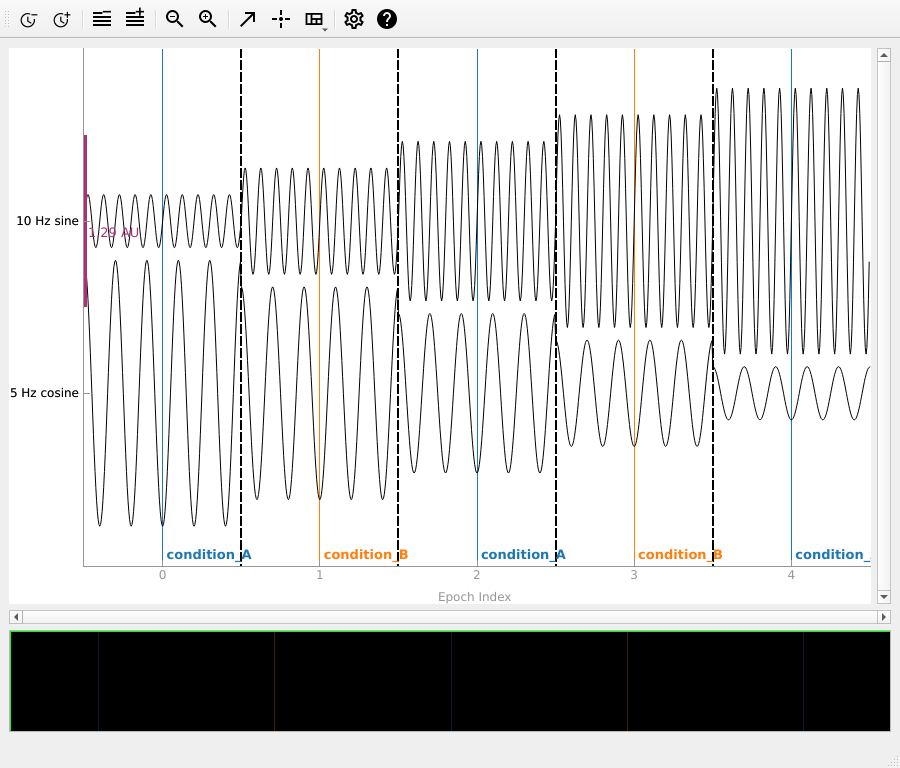```Not setting metadata
5 matching events found
No baseline correction applied
0 projection items activated
```

You could also create simulated epochs by using the normal `Epochs` (not `EpochsArray`) constructor on the simulated `RawArray` object, by creating an events array (e.g., using `mne.make_fixed_length_events`) and extracting epochs around those events.

## Creating `Evoked` Objects#

If you already have data that was averaged across trials, you can use it to create an `Evoked` object using the `EvokedArray` class constructor. It requires an `Info` object and a data array of shape `(n_channels, n_times)`, and has an optional `tmin` parameter like `EpochsArray` does. It also has a parameter `nave` indicating how many trials were averaged together, and a `comment` parameter useful for keeping track of experimental conditions, etc. Here we’ll do the averaging on our NumPy array and use the resulting averaged data to make our `Evoked`.

```# Create the Evoked object
evoked_array = mne.EvokedArray(
data.mean(axis=0), info, tmin=-0.5, nave=data.shape, comment="simulated"
)
print(evoked_array)
evoked_array.plot()
```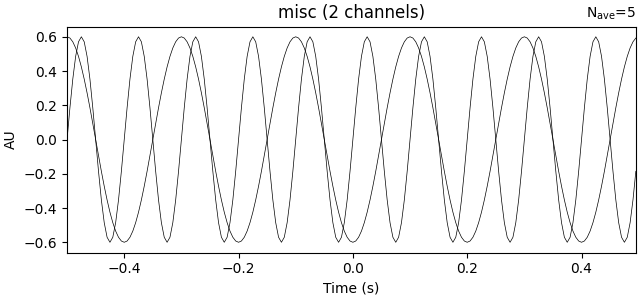```<Evoked | 'simulated' (average, N=5), -0.5 – 0.495 s, baseline off, 2 ch, ~10 kB>
```

In certain situations you may wish to use a custom time-frequency decomposition for estimation of power spectra. Or you may wish to process pre-computed power spectra in MNE. Following the same logic, it is possible to instantiate averaged power spectrum using the `Spectrum` class. This is slightly experimental at the moment but works. An API for doing this may follow.

```# compute power spectrum

psd, freqs = mne.time_frequency.psd_array_welch(
data, info["sfreq"], n_fft=128, n_per_seg=32
)

psd_ave = psd.mean(0)

# map to `~mne.time_frequency.Spectrum` class and explore API

def spectrum_from_array(
data: np.ndarray,  # spectral features
freqs: np.ndarray,  # frequencies
inst_info: mne.Info,  # the meta data of MNE instance
) -> mne.time_frequency.Spectrum:  # Spectrum object
"""Create MNE averaged power spectrum object from custom data"""
state = dict(
method="my_welch",
data=data,
sfreq=inst_info["sfreq"],
dims=("channel", "freq"),
freqs=freqs,
inst_type_str="Raw",
data_type="Averaged Power Spectrum",
info=inst_info,
)
defaults = dict(
method=None,
fmin=None,
fmax=None,
tmin=None,
tmax=None,
picks=None,
proj=None,
reject_by_annotation=None,
n_jobs=None,
verbose=None,
)
return mne.time_frequency.Spectrum(state, **defaults)

spectrum = spectrum_from_array(data=psd_ave, freqs=freqs, inst_info=info)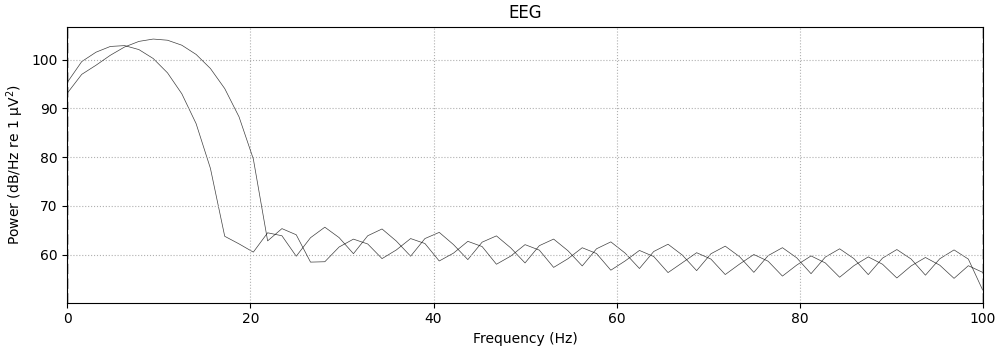```Effective window size : 0.640 (s)